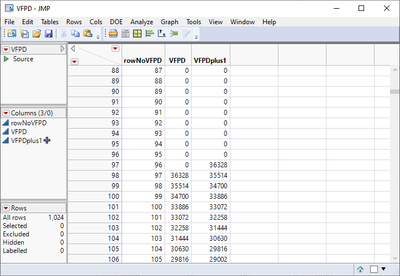Choose Language Hide Translation Bar
Highlighted

## Joining Tables with Formulae

I'm trying to join this table (below) to another by matching the column rowNoVFPD to one in another table.The column VFPDplus1 is assigned using the formula:

``Lag(:VFPD, -1).``

When I try to do this with JSL the VFPDplus1 column copies across blank, but otherwise correctly.
When I try using the JMP "Join" option in the "Table" toolbar it copies across correctly.

How can I solve this in JSL?

My current join function is written:

``````Data Table( "markTableSubset" ) << Join(
with( Data Table( "VFPD" ) ),
by matching columns( :FloorVdiv64 = :rowNoVFPD )
);``````

1 ACCEPTED SOLUTION

Accepted Solutions
Highlighted

## Re: Joining Tables with Formulae

I found my issue after a few weeks away to clear my head.

Here's an example snippet from my original script:

``````If( File Exists( path ), //Check file exits, import VFPD file
vfpdTable = Open( path, Import Settings( Data Starts( 5 ) ) );
Column( 1 ) << Data Type( Numeric ) << Modeling Type( "Continuous" ) << Set Name( "rowNoVFPD" );
Column( 2 ) << Set Name( "VFPD" );
New Column( "VFPDplus1", Numeric, Continuous, Formula (Lag(:VFPD,(-1)))); //Look at next row for interpolation after joining
Column( 3 ) << delete property(formula); //Copying formula causes issues when joining
);``````

My tables could exceed 500,000+ rows as they are large experimental data sets, so the formula had not finished calculating the results for the full column by the time I delete the formula property (a throw back to one of my original attempts to get the join to work), thus was not applying any of the formula results at all before joining. The same thing happened if I tried to join immediately after creating the new formula column.

I since changed the script to add a 1 second delay to let the formula calculate fully, and I can now join without issue. I hope this helps anyone else that comes across the same problem.

``````If( File Exists( path ), //Check file exits, import VFPD file
vfpdTable = Open( path, Import Settings( Data Starts( 5 ) ) );
Column( 1 ) << Data Type( Numeric ) << Modeling Type( "Continuous" ) << Set Name( "rowNoVFPD" );
Column( 2 ) << Set Name( "VFPD" );
New Column( "VFPDplus1", Numeric, Continuous, Formula (Lag(:VFPD,(-1)))); //Look at next row for interpolation after joining
Wait(1); //Necessary to stop deleting the formula before it's calculated the full column
Column( 3 ) << delete property(formula); //Copying formula causes issues when joining
);``````
3 REPLIES 3
Highlighted

## Re: Joining Tables with Formulae

Not sure why this would be but it might be because the order of rows in your "with" table is changed by the join.

Have you tried using the "Source" script that is generated in your joined table when you do the join through the tables menu?

I think that some of the optional arguments for the Join function, like "Preserve main table order" and "Copy formula", will be important.
Highlighted

## Re: Joining Tables with Formulae

I found my issue after a few weeks away to clear my head.

Here's an example snippet from my original script:

``````If( File Exists( path ), //Check file exits, import VFPD file
vfpdTable = Open( path, Import Settings( Data Starts( 5 ) ) );
Column( 1 ) << Data Type( Numeric ) << Modeling Type( "Continuous" ) << Set Name( "rowNoVFPD" );
Column( 2 ) << Set Name( "VFPD" );
New Column( "VFPDplus1", Numeric, Continuous, Formula (Lag(:VFPD,(-1)))); //Look at next row for interpolation after joining
Column( 3 ) << delete property(formula); //Copying formula causes issues when joining
);``````

My tables could exceed 500,000+ rows as they are large experimental data sets, so the formula had not finished calculating the results for the full column by the time I delete the formula property (a throw back to one of my original attempts to get the join to work), thus was not applying any of the formula results at all before joining. The same thing happened if I tried to join immediately after creating the new formula column.

I since changed the script to add a 1 second delay to let the formula calculate fully, and I can now join without issue. I hope this helps anyone else that comes across the same problem.

``````If( File Exists( path ), //Check file exits, import VFPD file
vfpdTable = Open( path, Import Settings( Data Starts( 5 ) ) );
Column( 1 ) << Data Type( Numeric ) << Modeling Type( "Continuous" ) << Set Name( "rowNoVFPD" );
Column( 2 ) << Set Name( "VFPD" );
New Column( "VFPDplus1", Numeric, Continuous, Formula (Lag(:VFPD,(-1)))); //Look at next row for interpolation after joining
Wait(1); //Necessary to stop deleting the formula before it's calculated the full column
Column( 3 ) << delete property(formula); //Copying formula causes issues when joining
);``````
Highlighted

## Re: Joining Tables with Formulae

Great. That makes sense. I'm glad you found a solution.
Phil
Article Labels

There are no labels assigned to this post.